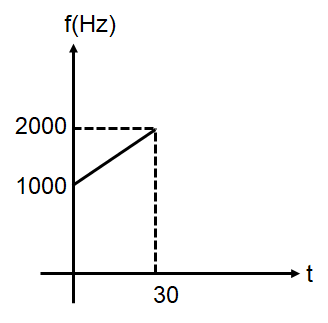Doppler
Question

# A detector is released from rest under gravity over a source of sound of frequency ${f}_{0}={10}^{3}Hz$ . The frequency observed by the detector at time t is plotted in the graph. The speed of sound in air is  $\left(g=10m/{\mathrm{sec}}^{2}\right)$:Easy
Solution

## $\mathrm{f}=\left(\frac{{\mathrm{V}}_{\mathrm{s}}+{\mathrm{V}}_{0}}{{\mathrm{V}}_{\mathrm{s}}}\right){\mathrm{f}}_{0}⇒\mathrm{f}=\left(\frac{{\mathrm{V}}_{\mathrm{s}}+\mathrm{gt}}{{\mathrm{V}}_{\mathrm{s}}}\right){\mathrm{f}}_{0}={\mathrm{f}}_{0}+\frac{{\mathrm{gf}}_{0}}{{\mathrm{V}}_{\mathrm{s}}}\mathrm{t}$

Get Instant Solutions